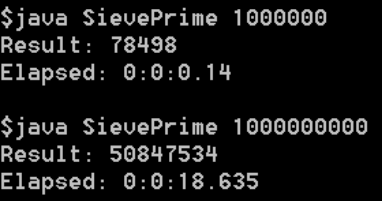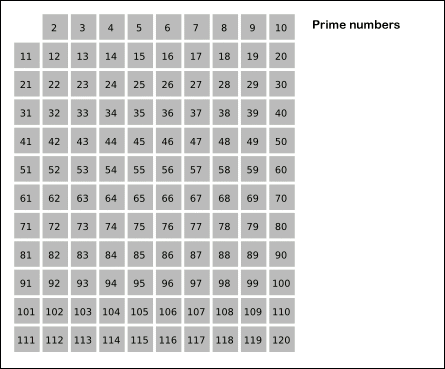# Counting Prime Numbers: Sieve of Eratosthenes using Java

New results:Old results:
CountPrime 5000 = 669 (Result in 10 seconds)
CountPrime 10000 = 1229 (Result in 85 seconds)

Last week I described here how to count prime numbers in a range using a rather unorthodox method called Hardy-Wright method. But as that method involves calculating factorials of all the numbers in the range [3..n], it is rather slow even when using multi-threading using a 8 core CPU.

So this week I was looking for a fast method to do this thing. I googled a bit and came across a method called Sieve of Eratosthenes.

## Part 1: Sieve of Eratosthenes

The sieve of Eratosthenes is one of the most efficient ways to find all of the smaller primes. It is named after Eratosthenes of Cyrene, a Greek mathematician.

To find all the prime numbers less than or equal to a given integer n by Eratosthenes’ method:

1. Create a list of consecutive integers from 2 through n: (2, 3, 4, …, n).
2. Initially, let p equal 2, the smallest prime number.
3. Enumerate the multiples of p by counting to n from 2p in increments of p, and mark them in the list (these will be 2p, 3p, 4p, … ; the p itself should not be marked).
4. Find the first number greater than p in the list that is not marked. If there was no such number, stop. Otherwise, let p now equal this new number (which is the next prime), and repeat from step 3.

As a refinement, it is sufficient to mark the numbers in step 3 starting from p2, as all the smaller multiples of p will have already been marked at that point. This means that the algorithm is allowed to terminate in step 4 when p2 is greater than n.Sieve of Eratosthenes: algorithm steps for primes below 121 (including optimization of starting from prime’s square).

## Part 2: The Algorithm

The sieve of Eratosthenes can be expressed in pseudo-code, as follows:

Input: an integer n > 1
Let A be an array of Boolean values, indexed by integers 2 to n, initially all set to true.
for i = 2, 3, 4, …, not exceeding √n:
if A[i] is true:
for j = i2, i2+i, i2+2i, i2+3i, …, not exceeding n :
A[j] := false
Output: all i such that A[i] is true.

So now we build a java program to execute this algorithm.

Please note the comments in the code to relate to the algorithm.

First we declare an array of Boolean, indexed from 0 to n, initially all set to true. We’ll not consider the first 2 elements while getting the output.

```private boolean isPrime[]; public SievePrime(int inp) { this.tempMax = inp; // Input: an integer n > 1 isPrime = new boolean[tempMax + 1]; // A be an array of Boolean values for (int i = 0; i <= tempMax; i++) { isPrime[i] = true; // initially all set to true } }```

Then we calculate the part “not exceeding √n” followed by the nested for loops to count the prime.

```int sqrtLimit = (int)Math.sqrt(tempMax); for (int i = tempMin; i <= sqrtLimit; i++) { if(isPrime[i]) { // for j = i2, i2+i, i2+2i, i2+3i, ..., not exceeding n : ctr = 0; mul = ((i * i) + (ctr * i)); while(mul <= tempMax) { isPrime[mul] = false; // A[j] := false ctr++; mul = ((i * i) + (ctr * i)); } } }```

Then we get the Output: all i such that A[i] is true.

``` // tempMin is 2, we are discarding the first two elements for(int i = tempMin; i <= tempMax; i++) { if(isPrime[i]) finalCount++; } System.out.println("Result: " + finalCount);```

## Part 3: Test and Benchmark

Alright, so that now we’ve built our program, it’s time to test it and get the timings. You can verify the results from here. And below is a screenshot of outputs of up to 1 billion.As you can see we could find primes till 1 million in well below the 1 second mark. Things only got slower for the input of 1 billion which took almost 19 seconds to complete. Not bad though.

1.